# ICSE Class 10 Physics Syllabus 2023-24: Download Class 10th Physics Syllabus PDF

0
475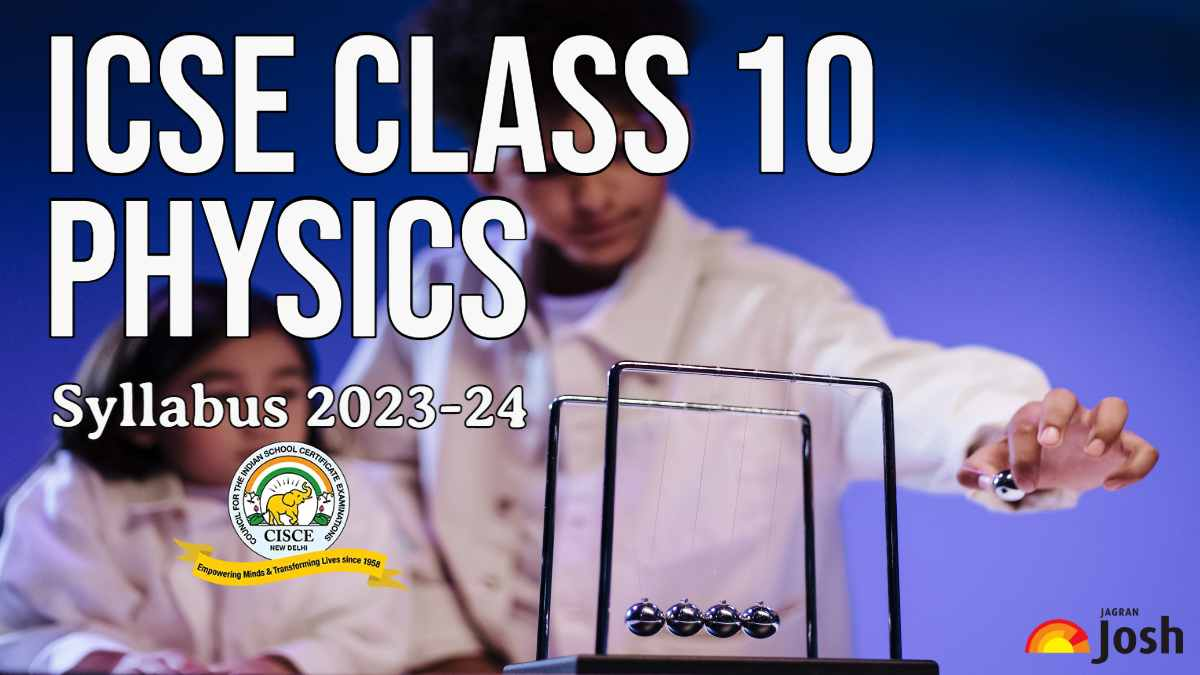ICSE Class 10 Physics Syllabus 2024: Get here the updated and detailed syllabus for ICSE Physics Class 10. Students can download the 2023-24 unit-wise syllabus pdf here.

ICSE Physics Syllabus for Class 10: CISCE (Council for the Indian School Certificate Examinations) is a well-known examination board in India. Every year it organises ICSE and ISC examinations all over the nation to check the validity of the knowledge students grabbed throughout their academic year. Well, the new academic session 2023-24 has just begun and it’s time for ICSE students to check the syllabus of all their subjects covered in class 10. A syllabus is a document that outlines the units/chapters and topics that will be covered in a specific subject. CISCE has released the subject-wise syllabus for ICSE 9 and 10 together. This article discusses the detailed syllabus of Physics, one of the components of science (52). Students must check and read the syllabus thoroughly along with additional guidelines to make things clearer.

Students can also check the note of SI units at the tail of this article. These basic notes will help you understand Physics better as it involves numerous conversions and the use of different symbols.

## ICSE Physics Syllabus; Aims:

1. To acquire knowledge and understanding of the terms, facts, concepts, definitions, laws, principles and processes of Physics.
2. To develop skills in practical aspects of handling apparatus, recording observations and in drawing diagrams, graphs, etc.
3. To develop instrumental, communication, deductive and problem-solving skills.
4. To discover that there is a living and growing physics relevant to the modern age in which we live.

## ICSE Class 10 Physics General Paper Guidelines:

1. There will be one paper of two hours duration carrying 80 marks and an Internal Assessment of practical work carrying 20 marks.
2. The paper will be divided into two sections, Section I (40 marks) and Section II (40 marks).
3. Section I (compulsory) will contain short answer questions on the entire syllabus.
4. Section II will contain six questions. Candidates will be required to answer any four of these six questions.
5. Note: Unless otherwise specified, only SI Units are to be used while teaching and learning, as well as for answering questions.

## Internal Assessment Of Practical Work:

Candidates will be asked to carry out experiments for which instructions will be given. The experiments may be based on topics that are not included in the syllabus but theoretical knowledge will not be required. A candidate will be expected to be able to follow simple instructions, to take suitable readings and to present these readings in a systematic form. He/she may be required to exhibit his/her data graphically. Candidates will be expected to appreciate and use the concepts of least count, significant figures and elementary error handling.

Note: Teachers may design their own set of experiments, preferably related to the theory syllabus. A comprehensive list is suggested below:

1. Lever – There are many possibilities with a meter rule as a lever with a load (known or unknown) suspended from a point near one end (say left), the lever itself pivoted on a knife edge, use slotted weights suspended from the other (right) side for effort.

Determine the mass of a metre rule using a spring balance or by balancing it on a knife edge at some point away from the middle and a 50g weight on the other side. Next pivot (F) the metre rule at the 40cm, 50cm and 60cm mark, each time suspending a load L or the left end and effort E near the right end. Adjust E and or its position so that the rule is balanced. Tabulate the position of L, F and E and the magnitudes of L and E and the distances of load arm and effort arm. Calculate MA=L/E and VR = effort arm/load arm. It will be found that MA <VR in one case, MA=VR in another and MA>VR in the third case. Try to explain why this is so. Also try to calculate the real load and real effort in these cases.

2. Determine the VR and MA of a given pulley system.

3. Trace the course of different rays of light refracting through a rectangular glass slab at different angles of incidence, measure the angles of incidence, refraction and emergence. Also measure the lateral displacement.

4. Determine the focal length of a convex lens by (a) the distant object method and (b) using a needle and a plane mirror.

5. Determine the focal length of a convex lens by using two pins and formula f = uv/(u+v).

6. For a triangular prism, trace the course of rays passing through it, measure angles i1, i2, A and δ.Repeat for four different angles of incidence (say i1=400, 500, 600 and 700). Verify i1+ i2=A+δ and A = r1 + r2.

7. For a ray of light incident normally (i1=0) on one face of a prism, trace course of the ray. Measure the angle δ. Explain briefly. Do this for prisms with A=600, 450and 900.

8. Calculate the specific heat capacity of the material of the given calorimeter, from the temperature readings and masses of cold water, warm water and its mixture taken in the calorimeter.

9. Determination of specific heat capacity of a metal by method of mixtures.

10. Determination of specific latent heat of ice.

11. Using as simple electric circuit, verify Ohm’s law. Draw a graph and obtain the slope.

12. Set up model of household wiring including ring main circuit. Study the function of switches and fuses.

Get the complete syllabus from the link below:

## A note on SI Units

SI units (Systeme International d’Unites) were adopted internationally in 1968.

### Fundamental Units

The system has seven fundamental (or basic) units, one for each of the fundamental quantities.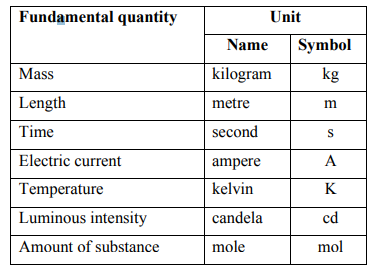### Derived units

These are obtained from the fundamental units by multiplication or division; no numerical factors are involved. Some derived units with complex names are: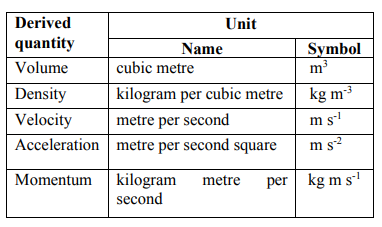Some derived units are given special names due to their complexity when expressed in terms of the fundamental units, as below: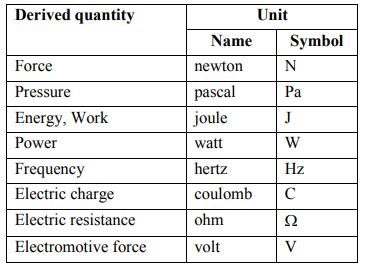When the unit is named after a person, the symbol has a capital letter.

### Standard prefixes

Decimal multiples and submultiples are attached to units when appropriate, as below: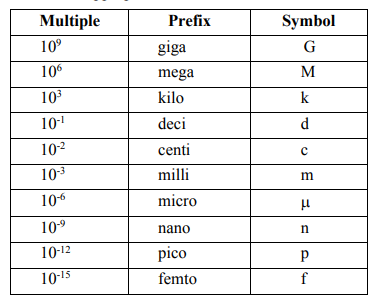Related:

SHARE